This post elaborates on the integration between Ray and Apache Arrow. The main problem this addresses is data serialization.

From Wikipedia, serialization is

… the process of translating data structures or object state into a format that can be stored … or transmitted … and reconstructed later (possibly in a different computer environment).

Why is any translation necessary? Well, when you create a Python object, it may have pointers to other Python objects, and these objects are all allocated in different regions of memory, and all of this has to make sense when unpacked by another process on another machine.

Serialization and deserialization are bottlenecks in parallel and distributed computing, especially in machine learning applications with large objects and large quantities of data.

## Design Goals

As Ray is optimized for machine learning and AI applications, we have focused a lot on serialization and data handling, with the following design goals:

1. It should be very efficient with large numerical data (this includes NumPy arrays and Pandas DataFrames, as well as objects that recursively contain Numpy arrays and Pandas DataFrames).
2. It should be about as fast as Pickle for general Python types.
3. It should be compatible with shared memory, allowing multiple processes to use the same data without copying it.
4. Deserialization should be extremely fast (when possible, it should not require reading the entire serialized object).
5. It should be language independent (eventually we’d like to enable Python workers to use objects created by workers in Java or other languages and vice versa).

## Our Approach and Alternatives

The go-to serialization approach in Python is the pickle module. Pickle is very general, especially if you use variants like cloudpickle. However, it does not satisfy requirements 1, 3, 4, or 5. Alternatives like json satisfy 5, but not 1-4.

Our Approach: To satisfy requirements 1-5, we chose to use the Apache Arrow format as our underlying data representation. In collaboration with the Apache Arrow team, we built libraries for mapping general Python objects to and from the Arrow format. Some properties of this approach:

• The data layout is language independent (requirement 5).
• Offsets into a serialized data blob can be computed in constant time without reading the full object (requirements 1 and 4).
• Arrow supports zero-copy reads, so objects can naturally be stored in shared memory and used by multiple processes (requirements 1 and 3).
• We can naturally fall back to pickle for anything we can’t handle well (requirement 2).

Alternatives to Arrow: We could have built on top of Protocol Buffers, but protocol buffers really isn’t designed for numerical data, and that approach wouldn’t satisfy 1, 3, or 4. Building on top of Flatbuffers actually could be made to work, but it would have required implementing a lot of the facilities that Arrow already has and we preferred a columnar data layout more optimized for big data.

## Speedups

Here we show some performance improvements over Python’s pickle module. The experiments were done using `pickle.HIGHEST_PROTOCOL`. Code for generating these plots is included at the end of the post.

With NumPy arrays: In machine learning and AI applications, data (e.g., images, neural network weights, text documents) are typically represented as data structures containing NumPy arrays. When using NumPy arrays, the speedups are impressive.

The fact that the Ray bars for deserialization are barely visible is not a mistake. This is a consequence of the support for zero-copy reads (the savings largely come from the lack of memory movement).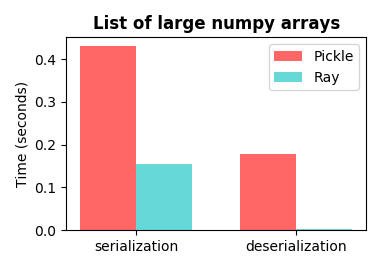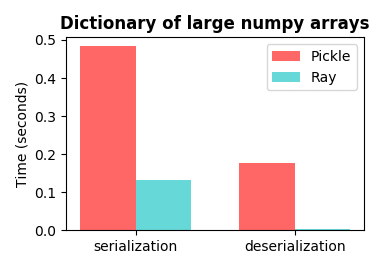Note that the biggest wins are with deserialization. The speedups here are multiple orders of magnitude and get better as the NumPy arrays get larger (thanks to design goals 1, 3, and 4). Making deserialization fast is important for two reasons. First, an object may be serialized once and then deserialized many times (e.g., an object that is broadcast to all workers). Second, a common pattern is for many objects to be serialized in parallel and then aggregated and deserialized one at a time on a single worker making deserialization the bottleneck.

Without NumPy arrays: When using regular Python objects, for which we cannot take advantage of shared memory, the results are comparable to pickle.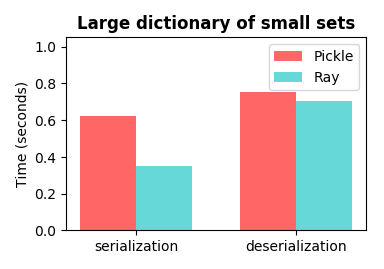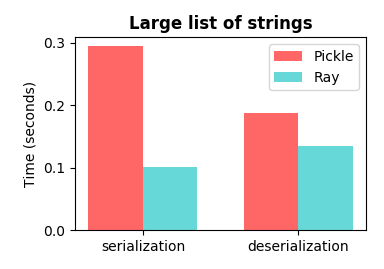These are just a few examples of interesting Python objects. The most important case is the case where NumPy arrays are nested within other objects. Note that our serialization library works with very general Python types including custom Python classes and deeply nested objects.

## The API

The serialization library can be used directly through pyarrow as follows. More documentation is available here.

``````x = [(1, 2), 'hello', 3, 4, np.array([5.0, 6.0])]
serialized_x = pyarrow.serialize(x).to_buffer()
deserialized_x = pyarrow.deserialize(serialized_x)
``````

It can be used directly through the Ray API as follows.

``````x = [(1, 2), 'hello', 3, 4, np.array([5.0, 6.0])]
x_id = ray.put(x)
deserialized_x = ray.get(x_id)
``````

## Data Representation

We use Apache Arrow as the underlying language-independent data layout. Objects are stored in two parts: a schema and a data blob. At a high level, the data blob is roughly a flattened concatenation of all of the data values recursively contained in the object, and the schema defines the types and nesting structure of the data blob.

Technical Details: Python sequences (e.g., dictionaries, lists, tuples, sets) are encoded as Arrow UnionArrays of other types (e.g., bools, ints, strings, bytes, floats, doubles, date64s, tensors (i.e., NumPy arrays), lists, tuples, dicts and sets). Nested sequences are encoded using Arrow ListArrays. All tensors are collected and appended to the end of the serialized object, and the UnionArray contains references to these tensors.

To give a concrete example, consider the following object.

``````[(1, 2), 'hello', 3, 4, np.array([5.0, 6.0])]
``````

It would be represented in Arrow with the following structure.

``````UnionArray(type_ids=[tuple, string, int, int, ndarray],
tuples=ListArray(offsets=[0, 2],
UnionArray(type_ids=[int, int],
ints=[1, 2])),
strings=['hello'],
ints=[3, 4],
ndarrays=[<offset of numpy array>])
``````

Arrow uses Flatbuffers to encode serialized schemas. Using only the schema, we can compute the offsets of each value in the data blob without scanning through the data blob (unlike Pickle, this is what enables fast deserialization). This means that we can avoid copying or otherwise converting large arrays and other values during deserialization. Tensors are appended at the end of the UnionArray and can be efficiently shared and accessed using shared memory.

Note that the actual object would be laid out in memory as shown below.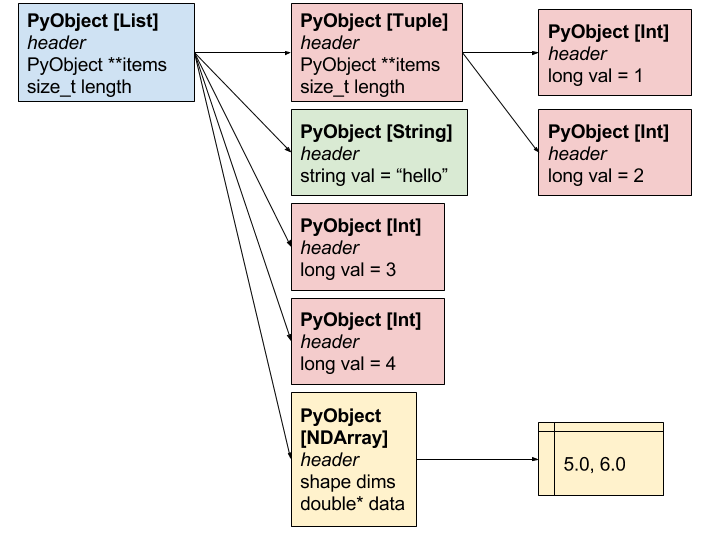The layout of a Python object in the heap. Each box is allocated in a different memory region, and arrows between boxes represent pointers.

The Arrow serialized representation would be as follows.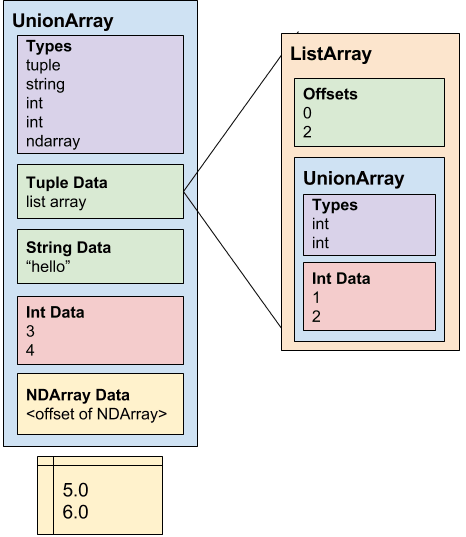The memory layout of the Arrow-serialized object.

## Getting Involved

We welcome contributions, especially in the following areas.

• Use the C++ and Java implementations of Arrow to implement versions of this for C++ and Java.
• Implement support for more Python types and better test coverage.

## Reproducing the Figures Above

For reference, the figures can be reproduced with the following code. Benchmarking `ray.put` and `ray.get` instead of `pyarrow.serialize` and `pyarrow.deserialize` gives similar figures. The plots were generated at this commit.

``````import pickle
import pyarrow
import matplotlib.pyplot as plt
import numpy as np
import timeit

def benchmark_object(obj, number=10):
# Time serialization and deserialization for pickle.
pickle_serialize = timeit.timeit(
lambda: pickle.dumps(obj, protocol=pickle.HIGHEST_PROTOCOL),
number=number)
serialized_obj = pickle.dumps(obj, pickle.HIGHEST_PROTOCOL)
number=number)

# Time serialization and deserialization for Ray.
ray_serialize = timeit.timeit(
lambda: pyarrow.serialize(obj).to_buffer(), number=number)
serialized_obj = pyarrow.serialize(obj).to_buffer()
ray_deserialize = timeit.timeit(
lambda: pyarrow.deserialize(serialized_obj), number=number)

return [[pickle_serialize, pickle_deserialize],
[ray_serialize, ray_deserialize]]

def plot(pickle_times, ray_times, title, i):
fig, ax = plt.subplots()
fig.set_size_inches(3.8, 2.7)

bar_width = 0.35
index = np.arange(2)
opacity = 0.6

plt.bar(index, pickle_times, bar_width,
alpha=opacity, color='r', label='Pickle')

plt.bar(index + bar_width, ray_times, bar_width,
alpha=opacity, color='c', label='Ray')

plt.title(title, fontweight='bold')
plt.ylabel('Time (seconds)', fontsize=10)
labels = ['serialization', 'deserialization']
plt.xticks(index + bar_width / 2, labels, fontsize=10)
plt.legend(fontsize=10, bbox_to_anchor=(1, 1))
plt.tight_layout()
plt.yticks(fontsize=10)
plt.savefig('plot-' + str(i) + '.png', format='png')

test_objects = [
[np.random.randn(50000) for i in range(100)],
{'weight-' + str(i): np.random.randn(50000) for i in range(100)},
{i: set(['string1' + str(i), 'string2' + str(i)]) for i in range(100000)},
[str(i) for i in range(200000)]
]

titles = [
'List of large numpy arrays',
'Dictionary of large numpy arrays',
'Large dictionary of small sets',
'Large list of strings'
]

for i in range(len(test_objects)):
plot(*benchmark_object(test_objects[i]), titles[i], i)
``````